2019独角兽企业重金招聘Python工程师标准>>>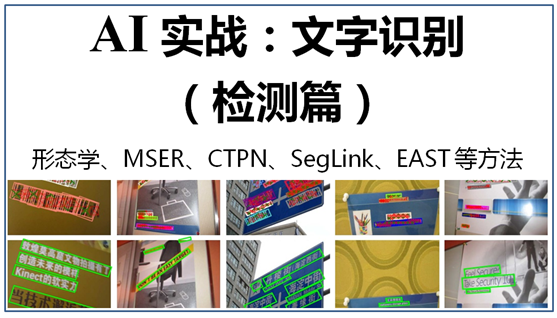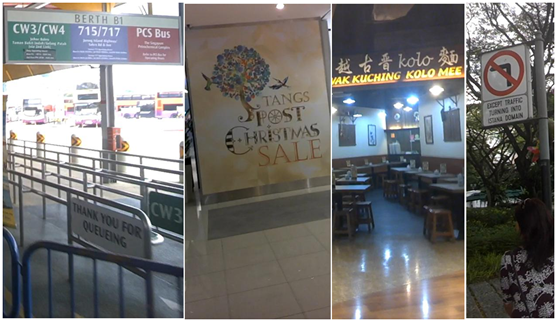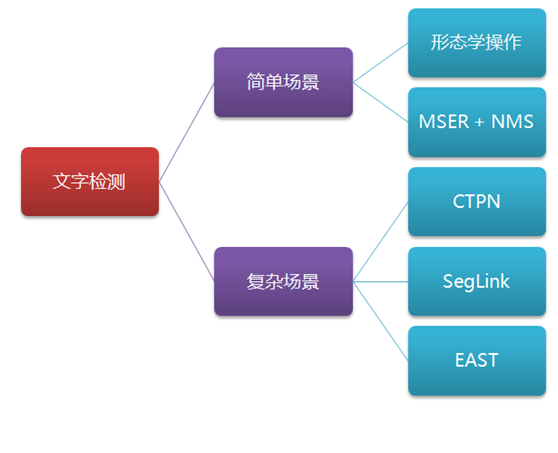1、简单场景：形态学操作法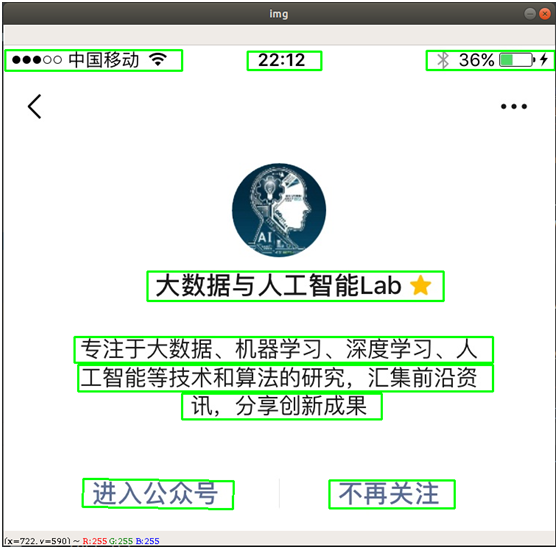• 读取图片，并转为灰度图
• 图片二值化，或先降噪后再二值化，以便简化处理
• 膨胀、腐蚀操作，突出轮廓、消除边框线条
• 查找轮廓，去除不符合文字特点的边框
• 返回文字检测的边框结果

# -*- coding: utf-8 -*-

import cv2
import numpy as np

# 读取图片

# 转化成灰度图
gray = cv2.cvtColor(img, cv2.COLOR_BGR2GRAY)

# 利用Sobel边缘检测生成二值图
sobel = cv2.Sobel(gray, cv2.CV_8U, 1, 0, ksize=3)
# 二值化
ret, binary = cv2.threshold(sobel, 0, 255, cv2.THRESH_OTSU + cv2.THRESH_BINARY)

# 膨胀、腐蚀
element1 = cv2.getStructuringElement(cv2.MORPH_RECT, (30, 9))
element2 = cv2.getStructuringElement(cv2.MORPH_RECT, (24, 6))

# 膨胀一次，让轮廓突出
dilation = cv2.dilate(binary, element2, iterations=1)

# 腐蚀一次，去掉细节
erosion = cv2.erode(dilation, element1, iterations=1)

# 再次膨胀，让轮廓明显一些
dilation2 = cv2.dilate(erosion, element2, iterations=2)

#  查找轮廓和筛选文字区域
region = []
contours, hierarchy = cv2.findContours(dilation2, cv2.RETR_TREE, cv2.CHAIN_APPROX_SIMPLE)
for i in range(len(contours)):
cnt = contours[i]

# 计算轮廓面积，并筛选掉面积小的
area = cv2.contourArea(cnt)
if (area < 1000):
continue

# 找到最小的矩形
rect = cv2.minAreaRect(cnt)
print ("rect is: ")
print (rect)

# box是四个点的坐标
box = cv2.boxPoints(rect)
box = np.int0(box)

# 计算高和宽
height = abs(box - box)
width = abs(box - box)

# 根据文字特征，筛选那些太细的矩形，留下扁的
if (height > width * 1.3):
continue

region.append(box)

# 绘制轮廓
for box in region:
cv2.drawContours(img, [box], 0, (0, 255, 0), 2)

cv2.imshow('img', img)
cv2.waitKey(0)
cv2.destroyAllWindows()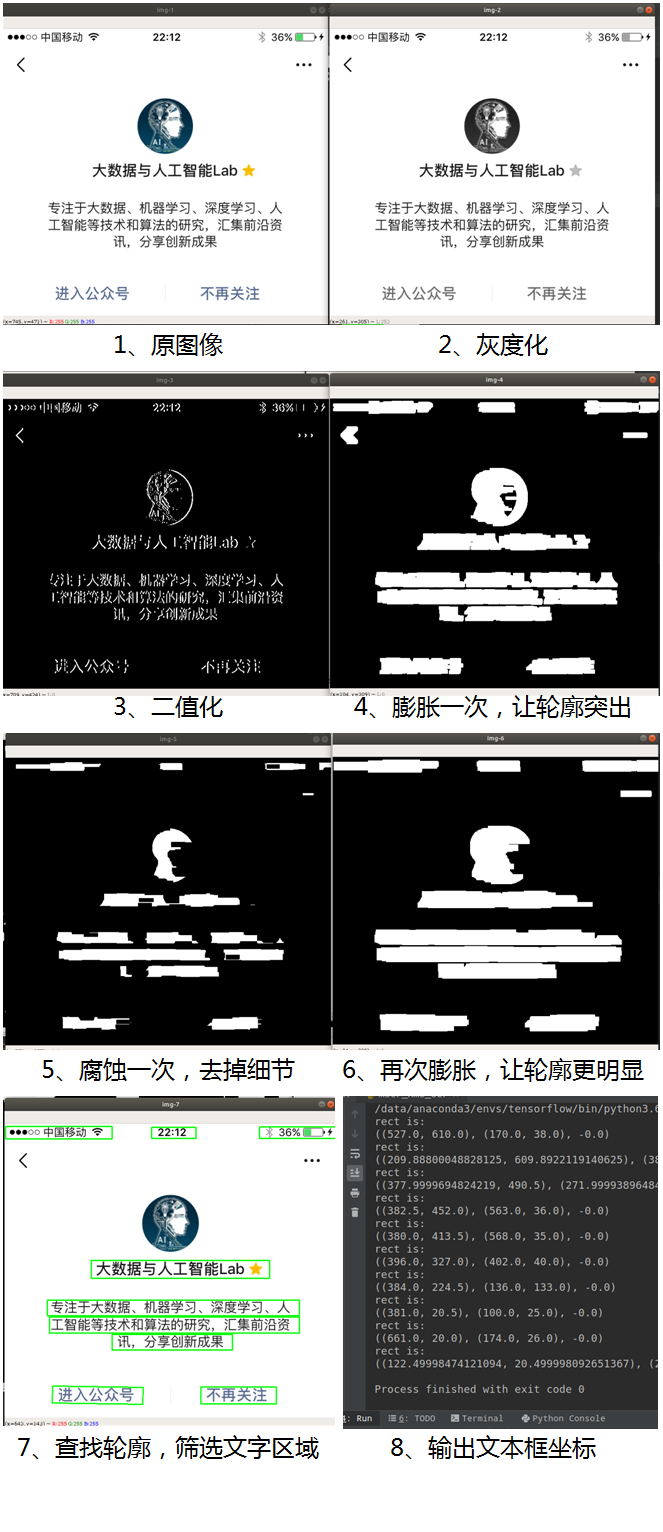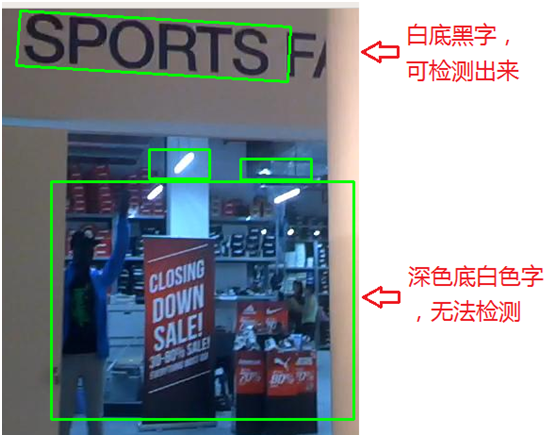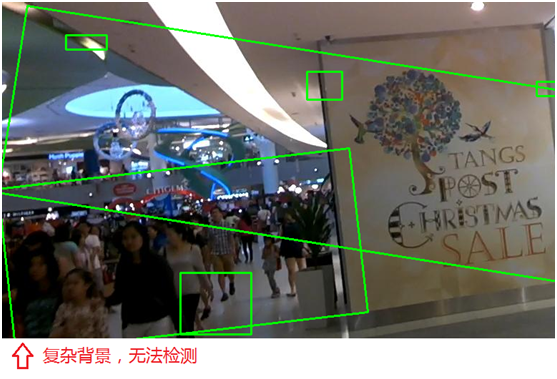2、简单场景：MSER+NMS检测法

MSER（Maximally Stable Extremal Regions，最大稳定极值区域）是一个较为流行的文字检测传统方法（相对于基于深度学习的AI文字检测而言），在传统OCR中应用较广，在某些场景下，又快又准。

MSER算法是在2002提出来的，主要是基于分水岭的思想进行检测。分水岭算法思想来源于地形学，将图像当作自然地貌，图像中每一个像素的灰度值表示该点的海拔高度，每一个局部极小值及区域称为集水盆地，两个集水盆地之间的边界则为分水岭，如下图：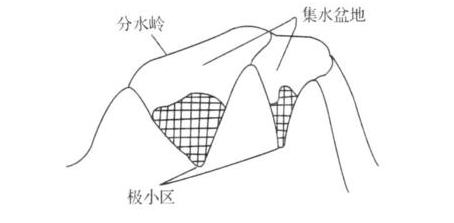MSER的处理过程是这样的，对一幅灰度图像取不同的阈值进行二值化处理，阈值从0至255递增，这个递增的过程就好比是一片土地上的水面不断上升，随着水位的不断上升，一些较低的区域就会逐渐被淹没，从天空鸟瞰，大地变为陆地、水域两部分，并且水域部分在不断扩大。在这个“漫水”的过程中，图像中的某些连通区域变化很小，甚至没有变化，则该区域就被称为最大稳定极值区域。在一幅有文字的图像上，文字区域由于颜色（灰度值）是一致的，因此在水平面（阈值）持续增长的过程中，一开始不会被“淹没”，直到阈值增加到文字本身的灰度值时才会被“淹没”。该算法可以用来粗略地定位出图像中的文字区域位置。# 读取图片

# 灰度化
gray = cv2.cvtColor(img, cv2.COLOR_BGR2GRAY)
vis = img.copy()
orig = img.copy()

# 调用 MSER 算法
mser = cv2.MSER_create()
regions, _ = mser.detectRegions(gray)  # 获取文本区域
hulls = [cv2.convexHull(p.reshape(-1, 1, 2)) for p in regions]  # 绘制文本区域
cv2.polylines(img, hulls, 1, (0, 255, 0))
cv2.imshow('img', img)

# 将不规则检测框处理成矩形框
keep = []
for c in hulls:
x, y, w, h = cv2.boundingRect(c)
keep.append([x, y, x + w, y + h])
cv2.rectangle(vis, (x, y), (x + w, y + h), (255, 255, 0), 1)
cv2.imshow("hulls", vis)


NMS算法的主要流程如下：

• 将所有框按置信度得分进行排序（如果边框没有置信度得分，也可以按坐标进行排序）
• 取其中得分最高的框出来
• 遍历该框与其余框的重叠面积（IoU）
• 删除IoU大于某个阈值的框（阈值可按需设定，例如0.3、0.5、0.8等）
• 取下一个得分最高的框出来，重复以上过程

# NMS 方法（Non Maximum Suppression，非极大值抑制）
def nms(boxes, overlapThresh):
if len(boxes) == 0:
return []

if boxes.dtype.kind == "i":
boxes = boxes.astype("float")

pick = []

# 取四个坐标数组
x1 = boxes[:, 0]
y1 = boxes[:, 1]
x2 = boxes[:, 2]
y2 = boxes[:, 3]

# 计算面积数组
area = (x2 - x1 + 1) * (y2 - y1 + 1)

# 按得分排序（如没有置信度得分，可按坐标从小到大排序，如右下角坐标）
idxs = np.argsort(y2)

# 开始遍历，并删除重复的框
while len(idxs) > 0:
# 将最右下方的框放入pick数组
last = len(idxs) - 1
i = idxs[last]
pick.append(i)

# 找剩下的其余框中最大坐标和最小坐标
xx1 = np.maximum(x1[i], x1[idxs[:last]])
yy1 = np.maximum(y1[i], y1[idxs[:last]])
xx2 = np.minimum(x2[i], x2[idxs[:last]])
yy2 = np.minimum(y2[i], y2[idxs[:last]])

# 计算重叠面积占对应框的比例，即 IoU
w = np.maximum(0, xx2 - xx1 + 1)
h = np.maximum(0, yy2 - yy1 + 1)
overlap = (w * h) / area[idxs[:last]]

# 如果 IoU 大于指定阈值，则删除
idxs = np.delete(idxs, np.concatenate(([last], np.where(overlap > overlapThresh))))

return boxes[pick].astype("int")MSER+NMS检测方法在传统的OCR应用中使用广泛，检测速度也非常快，能满足一定的文字识别场景。但当在复杂的自然场景中，特别是有复杂背景的，其检测效果也不尽人意，会将一些无关的因素也检测出来，如下图：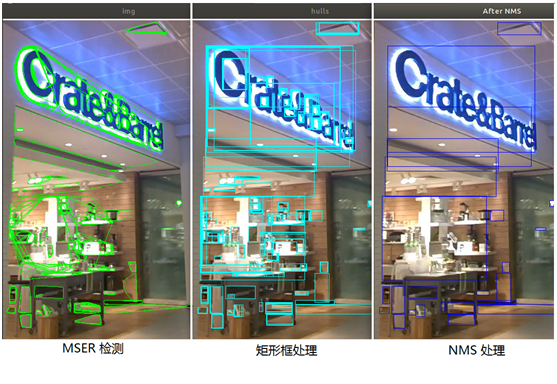【重点来了】

3、复杂场景：CTPN检测法

CTPN（Detecting Text in Natural Image with Connectionist Text Proposal Network，基于连接预选框网络的文本检测）是基于卷积神经网络和循环神经网络的文本检测方法，其基本做法是生成一系列适当尺寸的文本proposals（预选框）进行文本行的检测，示意图如下，具体的技术原理请见之前的文章（文章：大话文本检测经典模型：CTPN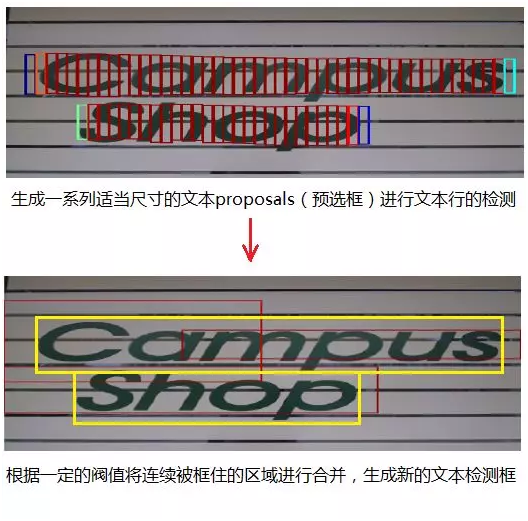CTPN检测法能适应较为复杂的自然场景，是目前深度学习中作文字检测的常用方法之一。CTPN的原作者提供该算法的源代码（https://github.com/tianzhi0549/CTPN），是基于caffe深度学习框架的。大家对tensorflow可能会更加熟悉，于是有人在github上提供了tensorflow版本的CTPN程序（https://github.com/eragonruan/text-detection-ctpn），下面介绍如何使用该程序进行文字检测。

（1）下载源代码和模型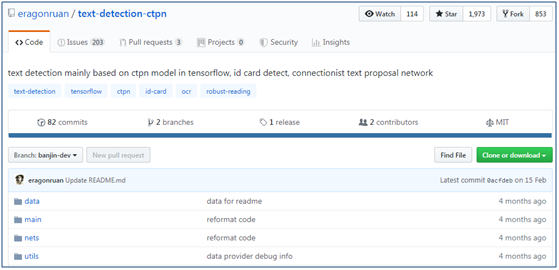① 首先，将tensorflow版本的CTPN程序源代码下载下来，可直接下载成zip压缩包或者git克隆

git clone https://github.com/eragonruan/text-detection-ctpn.git

② 接下来，进行编译安装，执行以下命令

cd utils/bbox

chmod +x make.sh

./make.sh

③ 下载预训练好的模型，下载地址为 https://pan.baidu.com/s/1BNHt_9fiqRPGmEXPaxaFXw ，下载后的压缩文件为checkpoints_mlt.zip，新建目录text-detection-ctpn，将解压后将 checkpoints_mlt 文件夹放到text-detection-ctpn 目录中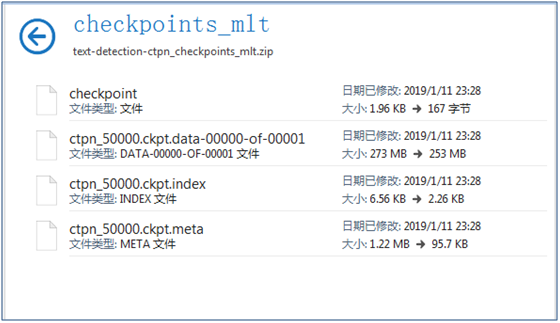（2）CTPN文本检测能力测试

python ./main/demo.py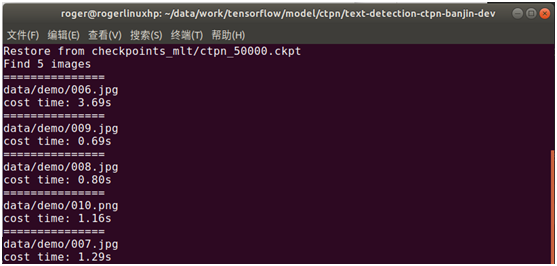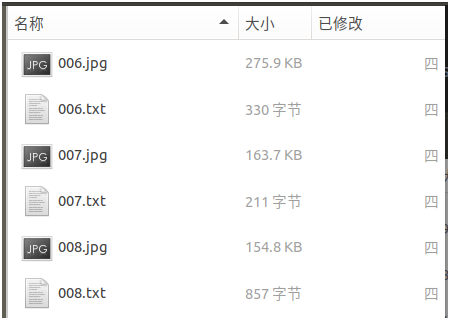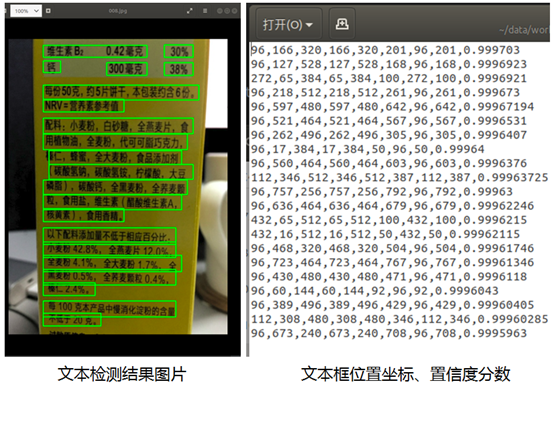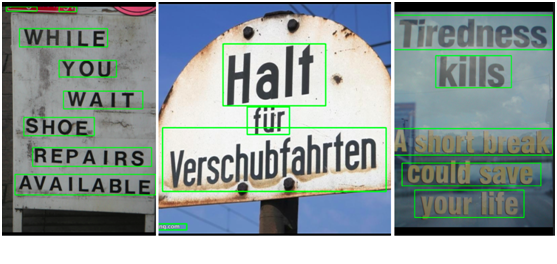（3）CTPN文本检测能力封装

# 基于 CTPN 的文字检测方法
# 输入：图片
# 返回：文本框位置和置信度分数
def text_detect(image):

with tf.get_default_graph().as_default():
# 模型参数定义
input_image = tf.placeholder(tf.float32, shape=[None, None, None, 3], name='input_image')
input_im_info = tf.placeholder(tf.float32, shape=[None, 3], name='input_im_info')

global_step = tf.get_variable('global_step', [], initializer=tf.constant_initializer(0), trainable=False)

bbox_pred, cls_pred, cls_prob = model.model(input_image)

variable_averages = tf.train.ExponentialMovingAverage(0.997, global_step)
saver = tf.train.Saver(variable_averages.variables_to_restore())

with tf.Session(config=tf.ConfigProto(allow_soft_placement=True)) as sess:
# 加载模型
ckpt_state = tf.train.get_checkpoint_state(checkpoint_dir)
model_path = os.path.join(checkpoint_dir, os.path.basename(ckpt_state.model_checkpoint_path))
saver.restore(sess, model_path)

# 预测文本框位置
img = image
h, w, c = img.shape
im_info = np.array([h, w, c]).reshape([1, 3])
bbox_pred_val, cls_prob_val = sess.run([bbox_pred, cls_prob],
feed_dict={input_image: [img],
input_im_info: im_info})

textsegs, _ = proposal_layer(cls_prob_val, bbox_pred_val, im_info)
scores = textsegs[:, 0]
textsegs = textsegs[:, 1:5]

textdetector = TextDetector(DETECT_MODE='H')
boxes = textdetector.detect(textsegs, scores[:, np.newaxis], img.shape[:2])
boxes = np.array(boxes, dtype=np.int)

return boxes,scores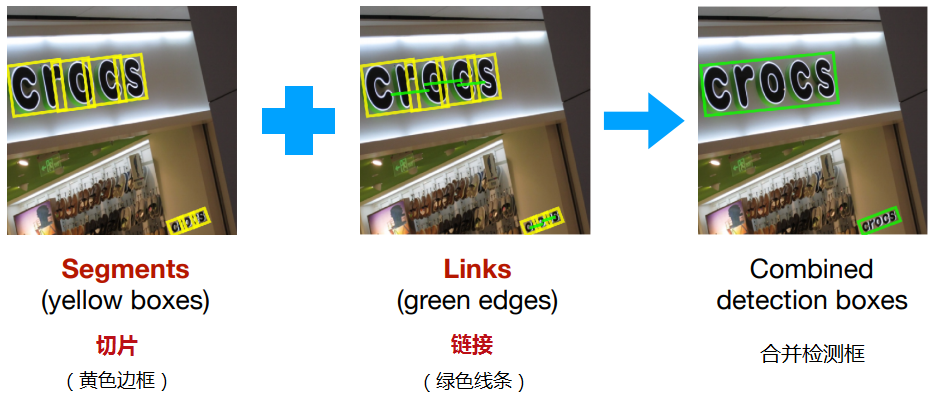（1）下载源代码和模型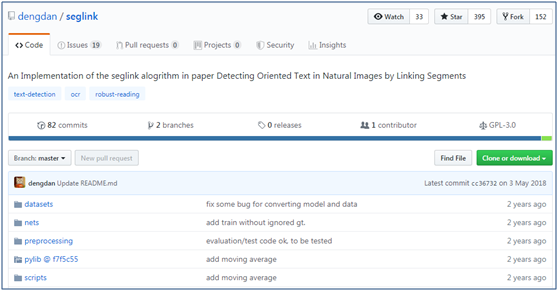git clone https://github.com/dengdan/seglink.git

import sys

sys.path.append('/data/PycharmProjects/tensorflow/ocr/seglink/util')

export PYTHONPATH=xx:\$PYTHONPATH

④ 安装依赖包

conda install -c cachemeorg setproctitle

#或以下命令

#pip install setproctitle

⑤ 如果python是使用了python3的，则需要进行以下修改（使用python 2.x的，请忽略）

• 修改pylib/util/io_.py，修改第11行，将import cPickle as pkl修改为import pickle as pkl
• 修改pylib/util/io_.py，修改第12行，将import commands改为import subprocess as commands
• 修改pylib/util/caffe_.py，修改第29行、第46行、第47行、第50行，在print后面加上括号
• 修改pylib/util/tf.py，修改第41行，将xrange改为range
• 修改config.py，修改第129行，将xrange改为range

# points = cv2.cv.BoxPoints(bbox)   #opencv2.4.9

points = cv2.boxPoints(bbox)       #opencv3.1.0

./scripts/test.sh 0 GPU_ID CKPT_PATH DATASET_DIR

./scripts/test.sh 0 ./models/seglink-512/model.ckpt-217867  ./dataset/ICDAR2015 /ch4_test_images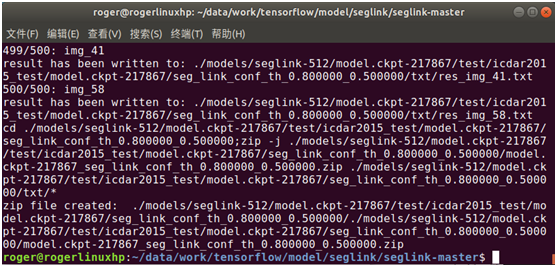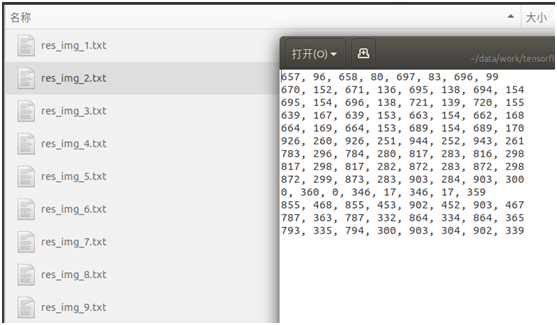python visualize_detection_result.py \

--image=检测的图片所在目录

--output=指定将文本框位置绘制到图片上的输出目录

① 在visualize_detection_result.py添加环境变量

import sys

sys.path.append('/data/PycharmProjects/tensorflow/ocr/seglink/util')

② 如果python是使用了python3的，则对visualize_detection_result.py第65行，print后面加上括号

python visualize_detection_result.py \

--image=./dataset/ICDAR2015/ ch4_test_images/  \

--output=./dataset/output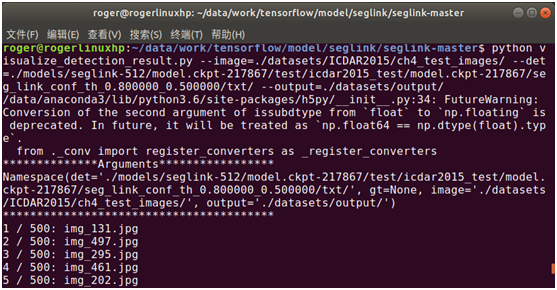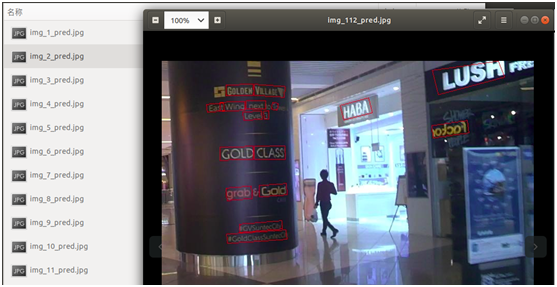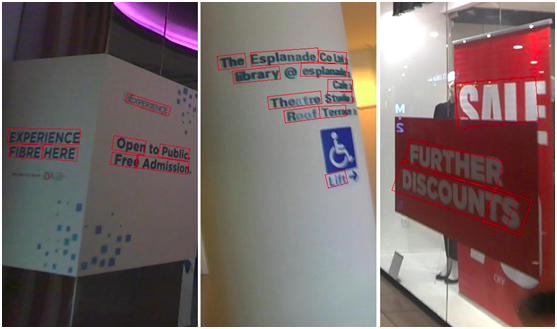# 基于 SegLink 的文字检测方法
# 输入：图片
# 返回：文本框位置
def text_detect(img):
with tf.name_scope('eval'):
with tf.variable_scope(tf.get_variable_scope(),reuse=True):
# 模型参数
image = tf.placeholder(dtype=tf.int32, shape=[None, None, 3])
image_shape = tf.placeholder(dtype=tf.int32, shape=[3, ])
# 预处理图片
processed_image, _, _, _, _ = ssd_vgg_preprocessing.preprocess_image(image, None, None, None, None,
out_shape=config.image_shape,
data_format=config.data_format,
is_training=False)
b_image = tf.expand_dims(processed_image, axis=0)
b_shape = tf.expand_dims(image_shape, axis=0)
# 预测文本框
net.seg_offsets,
image_shape=b_shape,
seg_conf_threshold=config.seg_conf_threshold,

sess_config = tf.ConfigProto(log_device_placement=False, allow_soft_placement=True)
sess_config.gpu_options.allow_growth = True

saver = tf.train.Saver()
if util.io.is_dir(checkpoint_dir):
checkpoint = util.tf.get_latest_ckpt(checkpoint_dir)
else:
checkpoint = checkpoint_dir

with tf.Session(config=sess_config) as sess:
# 加载模型
saver.restore(sess, checkpoint)
# 预测文本框
image_data = img
image_bboxes = sess.run([bboxes_pred], feed_dict={image: image_data, image_shape: image_data.shape})
bboxes = image_bboxes

return bboxes


5、复杂场景：EAST检测法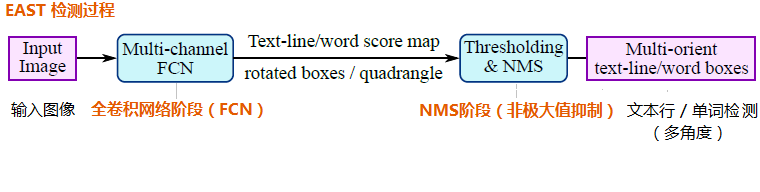（1）下载源代码和模型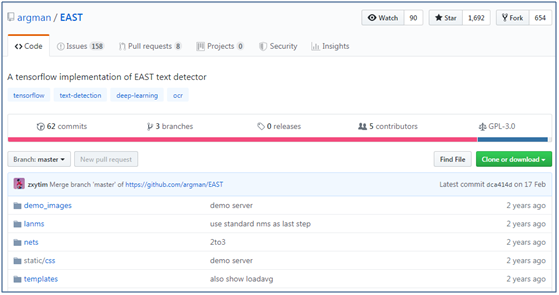① 首先在github上下载EAST的源代码（https://github.com/argman/EAST），可直接下载成zip压缩包或者git克隆

git clone https://github.com/argman/EAST.git

② 在百度网盘上下载预先训练好的模型文件（基于ICDAR 2013、ICDAR 2015数据集训练），下载地址为http://pan.baidu.com/s/1jHWDrYQ

③ 安装shapely依赖包，执行以下命令

conda install shapely

# 或执行以下命令

# pip install shapely

（2）EAST检测文本测试（demo页面）

python run_demo_server.py –checkpoint_path model/east_icdar2015_resnet_v1_50_rbox/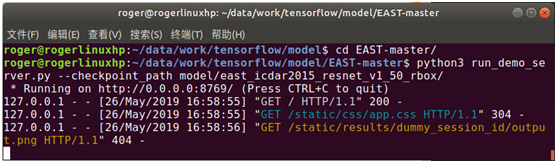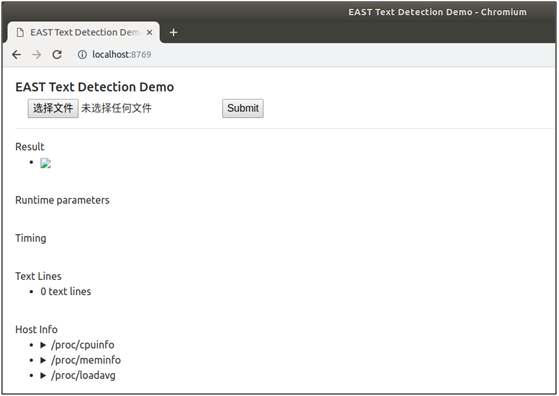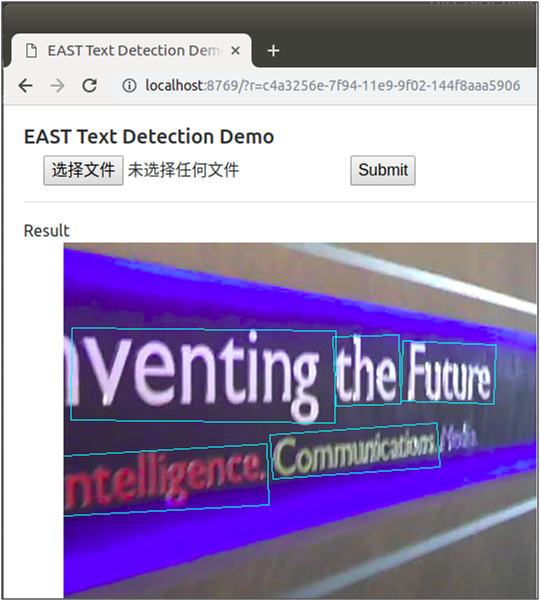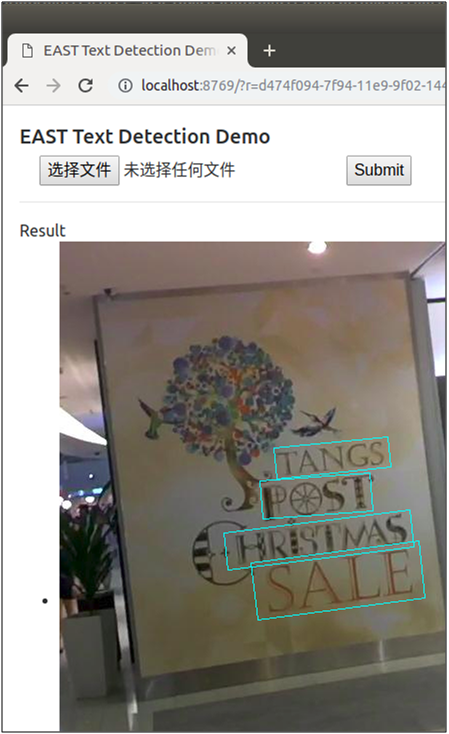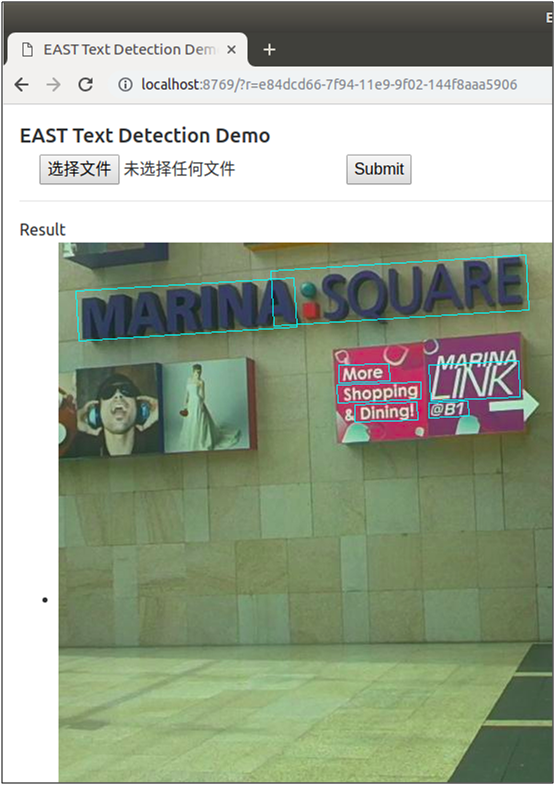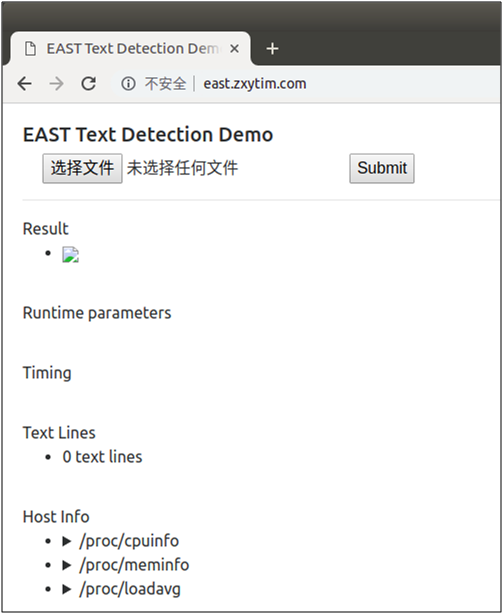（3）EAST检测文本测试（批量检测）

python eval.py –test_data_path=/data/work/tensorflow/model/seglink/ICDAR2015/ch4_test_images/ --checkpoint_path=model/east_icdar2015_resnet_v1_50_rbox/ --output_dir=/tmp/east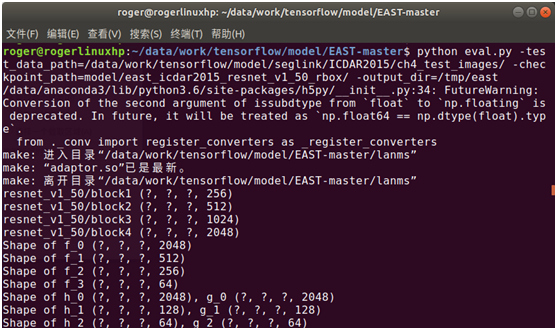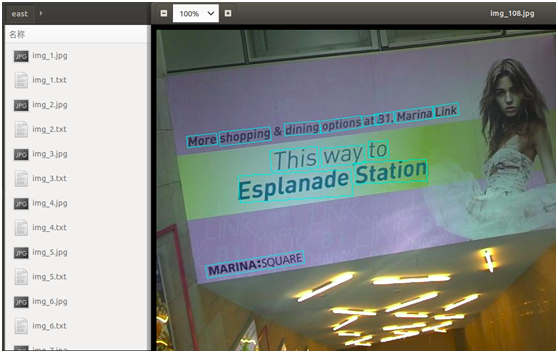（4）EAST文本检测能力封装

# 基于 EAST 的文字检测方法
# 输入：图片
# 返回：文本框位置相关信息
def text_detect(img):
# 模型路径
checkpoint_path='/data/PycharmProjects/tensorflow/ocr/east/model/east_icdar2015_resnet_v1_50_rbox/'

# 模型参数
input_images = tf.placeholder(tf.float32, shape=[None, None, None, 3], name='input_images')
global_step = tf.get_variable('global_step', [], initializer=tf.constant_initializer(0), trainable=False)

f_score, f_geometry = model.model(input_images, is_training=False)

variable_averages = tf.train.ExponentialMovingAverage(0.997, global_step)
saver = tf.train.Saver(variable_averages.variables_to_restore())

sess = tf.Session(config=tf.ConfigProto(allow_soft_placement=True))

# 加载模型
ckpt_state = tf.train.get_checkpoint_state(checkpoint_path)
model_path = os.path.join(checkpoint_path, os.path.basename(ckpt_state.model_checkpoint_path))
saver.restore(sess, model_path)

# 预测文本框
im_resized, (ratio_h, ratio_w) = resize_image(img)
score, geometry = sess.run(
[f_score, f_geometry],
feed_dict={input_images: [im_resized[:,:,::-1]]})

boxes,_ = detect(score_map=score, geo_map=geometry, timer=collections.OrderedDict([('net', 0),('restore', 0),('nms', 0)]))

if boxes is not None:
scores = boxes[:,8].reshape(-1)
boxes = boxes[:, :8].reshape((-1, 4, 2))
boxes[:, :, 0] /= ratio_w
boxes[:, :, 1] /= ratio_h

text_lines = []
if boxes is not None:
text_lines = []
for box, score in zip(boxes, scores):
box = sort_poly(box.astype(np.int32))
if np.linalg.norm(box - box) < 5 or np.linalg.norm(box-box) < 5:
continue
tl = collections.OrderedDict(zip(
['x0', 'y0', 'x1', 'y1', 'x2', 'y2', 'x3', 'y3'],
map(float, box.flatten())))
tl['score'] = float(score)
text_lines.append(tl)
ret = {
'text_lines': text_lines,
}
return ret1、AI 实战系列

2、大话深度学习系列

3、AI 杂谈

4、大数据超详细系列

06-10776
07-21
10-281万+
02-29512
02-1969
07-28134
11-301214
10-281466
12-173970
12-053455
05-11569
10-10167
05-1684
08-114498
06-18436
11-11687
06-17412
05-05119
06-08164

### “相关推荐”对你有帮助么？

•非常没帮助
•没帮助
•一般
•有帮助
•非常有帮助被折叠的  条评论 为什么被折叠?到【灌水乐园】发言点击重新获取扫码支付1.余额是钱包充值的虚拟货币，按照1:1的比例进行支付金额的抵扣。
2.余额无法直接购买下载，可以购买VIP、C币套餐、付费专栏及课程。余额充值